# How to use the REPLACE function in Excel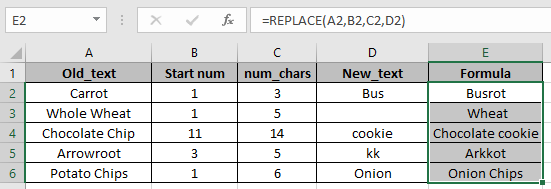In this article, we will learn How to use the ... function in Excel.

What is replace and substitute in Excel ?

There are two functions in excel which are used to replace value in already existing values. You must be thinking why Excel gives two similar functions for the same task. There is a huge difference in the usage of two functions.

SUBSTITUTE function substitutes value in place of other given value. For example replace "at" with "on" in text wherever existed or number of times to edit. These flexibilities are handled by SUBSTITUTE function. Unlike the REPLACE function we don't need to provide the position of the replacement text. Let's learn more about SUBSTITUTE function syntax and an example to illustrate its use.

REPLACE Function in Excel

The REPLACE function replaces the text from the cell. Replaced text is provided in the formula. Unlike SUBSTITUTE function we need to provide the position of the replacement text.

Syntax:

 =REPLACE (old_text, start_num, num_chars, new_text)

old_text is the find text

start_num is the first replacement begin from

num_chars is the the count of characters to replace beginning from start_num

new_text is the replace text

Example :

All of these might be confusing to understand. Let's understand how to use the function using an example. Here we have some Old_text and other arguments of the REPLACE function.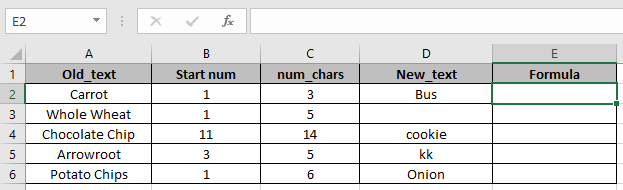Use the formula

 =REPLACE(A2,B2,C2,D2)

Explanation:

Starting from position 1

3 chars will be replaced with the new text Bus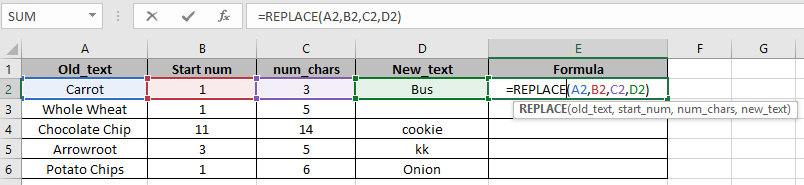Press Enter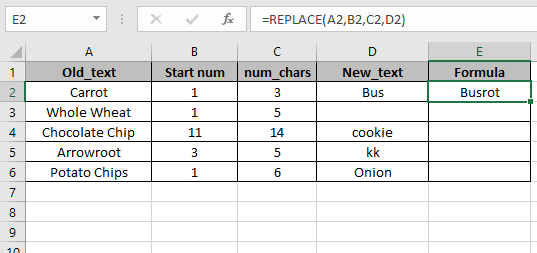Copy the formula in other cells, select the cells taking the first cell where the formula is already applied, use shortcut key Ctrl + DIn the E3 cell we just erased the text Whole from the A3 Cell.

SUBSTITUTE function and REPLACE function

Here we have the same text. We will perform multiple tasks on it.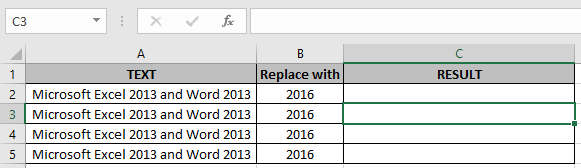Use Substitute function to replace 2013 to 2016 (First occurrence)

 =SUBSTITUTE(A2,2013,B2,1)

Use Replace function to replace 2013 to 2016 (First occurrence)

 =REPLACE(A3,17,4,B3)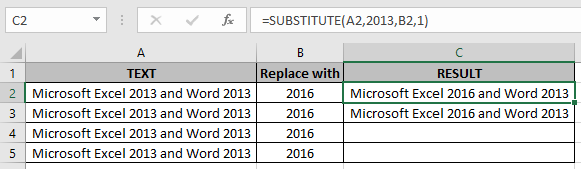Now we need to replace both 2013 text

Use Substitute function to replace 2013 to 2016 (All occurrence)

 =SUBSTITUTE(A2,2013,B2)

Use Replace function to replace 2013 to 2016 (All occurrence)

 =REPLACE(REPLACE(A5,17,4,B5),31,4,B5)

Nested REPLACE function replaces both texts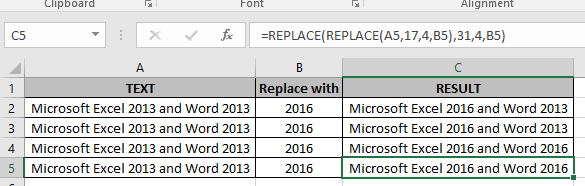The results are in front of you. As we can see text been changed.

Here are all the observational notes using the SUBSTITUTE function in Excel
Notes :

1. Use the SUBSTITUTE function, if you know the text to replace with new text in the old_text.
2. Be careful using the order of arguments in the function.

Hope this article about How to use the ... function in Excel is explanatory. Find more articles on calculating values and related Excel formulas here. If you liked our blogs, share it with your friends on Facebook. And also you can follow us on Twitter and Facebook. We would love to hear from you, do let us know how we can improve, complement or innovate our work and make it better for you. Write to us at info@exceltip.com.

Related Articles :

Excel REPLACE vs SUBSTITUTE function: The REPLACE and SUBSTITUTE functions are the most misunderstood functions. To find and replace a given text we use the SUBSTITUTE function. Where REPLACE is used to replace a number of characters in string…

How to Use TRIM function in Excel: The TRIM function is used to trim strings and clean any trailing or leading spaces from string. This helps us in the cleaning process of data a lot.

How to use the CLEAN function in Excel: Clean function is used to clean up unprintable characters from the string. This function is mostly used with the TRIM function to clean up imported foreign data.

Replace text from end of a string starting from variable position: To replace text from the end of the string, we use the REPLACE function. The REPLACE function uses the position of text in the string to replace.

How to Check if a cell contains one of many texts in Excel: To find check if a string contains any of multiple text, we use this formula. We use the SUM function to sum up all the matches and then perform a logic to check if the string contains any of the multiple strings.

Count Cells that contain specific text: A simple COUNTIF function will do the magic. To count the number of multiple cells that contain a given string we use the wildcard operator with the COUNTIF function.

Excel REPLACE vs SUBSTITUTE function: The REPLACE and SUBSTITUTE functions are the most misunderstood functions. To find and replace a given text we use the SUBSTITUTE function. Where REPLACE is used to replace a number of characters in string…

Popular Articles :

How to use the IF Function in Excel : The IF statement in Excel checks the condition and returns a specific value if the condition is TRUE or returns another specific value if FALSE.

How to use the VLOOKUP Function in Excel : This is one of the most used and popular functions of excel that is used to lookup value from different ranges and sheets.

How to use the SUMIF Function in Excel : This is another dashboard essential function. This helps you sum up values on specific conditions.

How to use the COUNTIF Function in Excel : Count values with conditions using this amazing function. You don't need to filter your data to count specific values. Countif function is essential to prepare your dashboard.

Terms and Conditions of use

The applications/code on this site are distributed as is and without warranties or liability. In no event shall the owner of the copyrights, or the authors of the applications/code be liable for any loss of profit, any problems or any damage resulting from the use or evaluation of the applications/code.